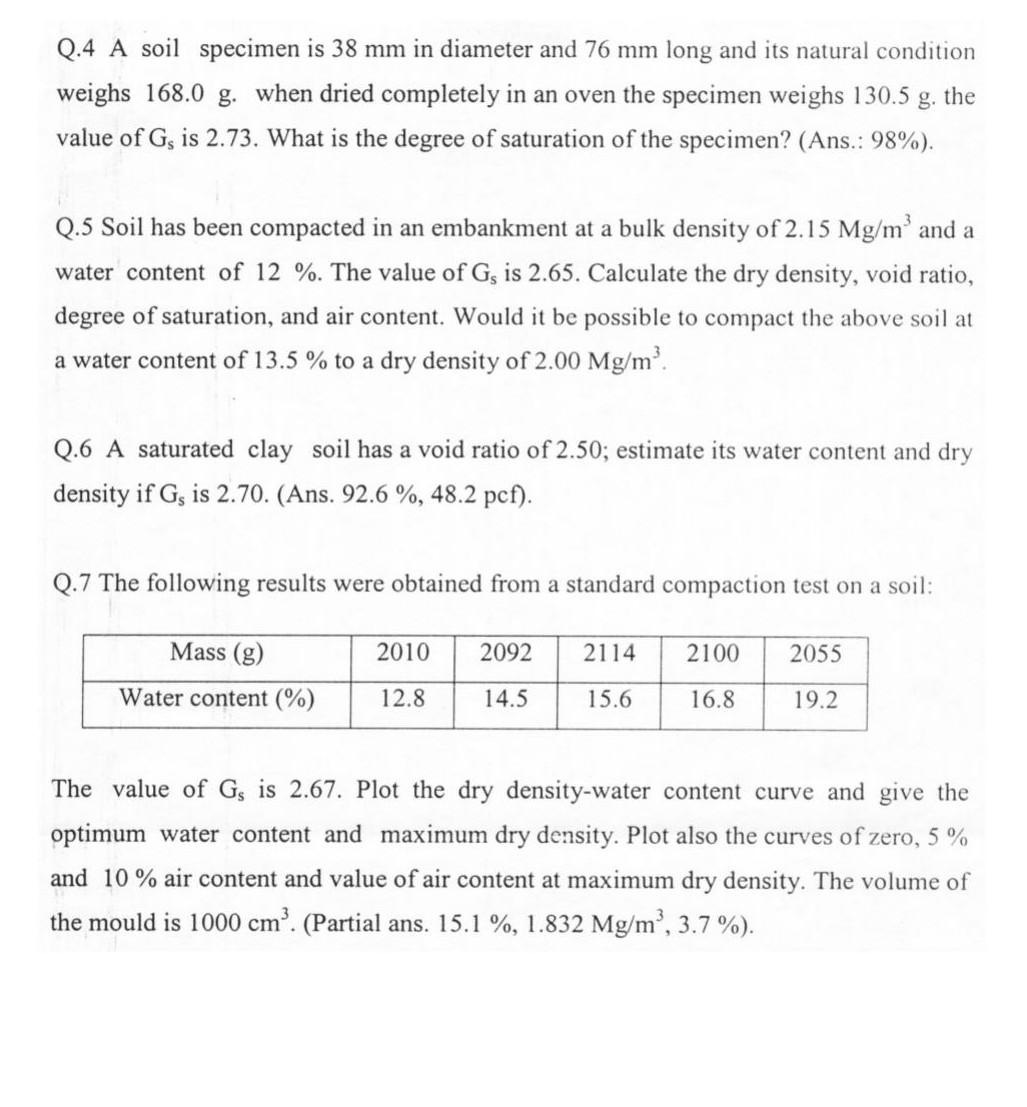# Question Solved1 AnswerQ.4 A soil specimen is 38 mm in diameter and 76 mm long and its natural condition weighs 168.0 g. when dried completely in an oven the specimen weighs 130.5 the value of G, is 2.73. What is the degree of saturation of the specimen? (Ans.: 98%). Q.5 Soil has been compacted in an embankment at a bulk density of 2.15 Mg/m' and a water content of 12 %. The value of Gs is 2.65. Calculate the dry density, void ratio, degree of saturation, and air content. Would it be possible to compact the above soil at a water content of 13.5 % to a dry density of 2.00 Mg/m'. Q.6 A saturated clay soil has a void ratio of 2.50; estimate its water content and dry density if Gs is 2.70. (Ans. 92.6 %, 48.2 pcf). Q.7 The following results were obtained from a standard compaction test on a soil: Mass (g) 2010 2092 2114 2100 2055 Water content (%) 12.8 14.5 15.6 16.8 19.2 The value of Gs is 2.67. Plot the dry density-water content curve and give the optimum water content and maximum dry density. Plot also the curves of zero, 5 % and 10 % air content and value of air content at maximum dry density. The volume of the mould is 1000 cm'. (Partial ans. 15.1 %, 1.832 Mg/m², 3.7%).VFFTEG The Asker · Civil EngineeringTranscribed Image Text: Q.4 A soil specimen is 38 mm in diameter and 76 mm long and its natural condition weighs 168.0 g. when dried completely in an oven the specimen weighs 130.5 the value of G, is 2.73. What is the degree of saturation of the specimen? (Ans.: 98%). Q.5 Soil has been compacted in an embankment at a bulk density of 2.15 Mg/m' and a water content of 12 %. The value of Gs is 2.65. Calculate the dry density, void ratio, degree of saturation, and air content. Would it be possible to compact the above soil at a water content of 13.5 % to a dry density of 2.00 Mg/m'. Q.6 A saturated clay soil has a void ratio of 2.50; estimate its water content and dry density if Gs is 2.70. (Ans. 92.6 %, 48.2 pcf). Q.7 The following results were obtained from a standard compaction test on a soil: Mass (g) 2010 2092 2114 2100 2055 Water content (%) 12.8 14.5 15.6 16.8 19.2 The value of Gs is 2.67. Plot the dry density-water content curve and give the optimum water content and maximum dry density. Plot also the curves of zero, 5 % and 10 % air content and value of air content at maximum dry density. The volume of the mould is 1000 cm'. (Partial ans. 15.1 %, 1.832 Mg/m², 3.7%).
More
Transcribed Image Text: Q.4 A soil specimen is 38 mm in diameter and 76 mm long and its natural condition weighs 168.0 g. when dried completely in an oven the specimen weighs 130.5 the value of G, is 2.73. What is the degree of saturation of the specimen? (Ans.: 98%). Q.5 Soil has been compacted in an embankment at a bulk density of 2.15 Mg/m' and a water content of 12 %. The value of Gs is 2.65. Calculate the dry density, void ratio, degree of saturation, and air content. Would it be possible to compact the above soil at a water content of 13.5 % to a dry density of 2.00 Mg/m'. Q.6 A saturated clay soil has a void ratio of 2.50; estimate its water content and dry density if Gs is 2.70. (Ans. 92.6 %, 48.2 pcf). Q.7 The following results were obtained from a standard compaction test on a soil: Mass (g) 2010 2092 2114 2100 2055 Water content (%) 12.8 14.5 15.6 16.8 19.2 The value of Gs is 2.67. Plot the dry density-water content curve and give the optimum water content and maximum dry density. Plot also the curves of zero, 5 % and 10 % air content and value of air content at maximum dry density. The volume of the mould is 1000 cm'. (Partial ans. 15.1 %, 1.832 Mg/m², 3.7%).